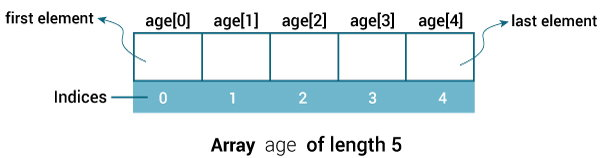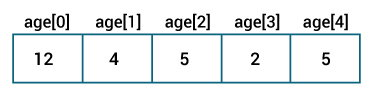# Java Arrays

In this article, you will learn to work with arrays in Java. You will learn to declare, initialize and, access array elements with the help of examples.

An array is a container that holds data (values) of one single type. For example, you can create an array that can hold 100 values of `int` type.

Array is a fundamental construct in Java that allows you to store and access large number of values conveniently.

## How to declare an array?

Here's how you can declare an array in Java:

```dataType[] arrayName;
```
• dataType can be a primitive data type like: int, char, Double, byte etc. or an object (will be discussed in later chapters).
• arrayName is an identifier.

Let's take the above example again.

`double[] data;`

Here, data is an array that can hold values of type `Double`.

But, how many elements can array this hold?

Good question! We haven't defined it yet. The next step is to allocate memory for array elements.

`data = new Double;`

The length of `data` array is 10. Meaning, it can hold 10 elements (10 `Double` values in this case).

Note, once the length of the array is defined, it cannot be changed in the program.

Let's take another example:

```int[] age;
age = new int;```

Here, age array can hold 5 values of type int.

It's possible to declare and allocate memory of an array in one statement. You can replace two statements above with a single statement.

`int[] age = new int;`

## Java Array Index

You can access elements of an array by using indices. Let's consider previous example.

`int[] age = new int;`The first element of array is `age`, second is `age` and so on.

If the length of an array is n, the last element will be `arrayName[n-1]`. Since the length of age array is 5, the last element of the array is `age` in the above example.

The default initial value of elements of an array is 0 for numeric types and `false` for `boolean`. We can demonstrate this:

``````class ArrayExample {
public static void main(String[] args) {

int[] age = new int;

System.out.println(age);
System.out.println(age);
System.out.println(age);
System.out.println(age);
System.out.println(age);
}
}```
```

When you run the program, the output will be:

```0
0
0
0
0
```

There is a better way to access elements of an array by using looping construct (generally for loop is used).

``````class ArrayExample {
public static void main(String[] args) {

int[] age = new int;

for (int i = 0; i < 5; ++i) {
System.out.println(age[i]);
}
}
}``````

## How to initialize arrays in Java?

In Java, you can initialize arrays during declaration or you can initialize (or change values) later in the program as per your requirement.

### Initialize an Array During Declaration

Here's how you can initialize an array during declaration.

`int[] age = {12, 4, 5, 2, 5};`

This statement creates an array and initializes it during declaration.

The length of the array is determined by the number of values provided which is separated by commas. In our example, the length of `age` array is 5.Let's write a simple program to print elements of this array.

``````class ArrayExample {
public static void main(String[] args) {

int[] age = {12, 4, 5, 2, 5};

for (int i = 0; i < 5; ++i) {
System.out.println("Element at index " + i +": " + age[i]);
}
}
}```
```

When you run the program, the output will be:

```Element at index 0: 12
Element at index 1: 4
Element at index 2: 5
Element at index 3: 2
Element at index 4: 5```

## How to access array elements?

You can easily access and alter array elements by using its numeric index. Let's take an example.

``````class ArrayExample {
public static void main(String[] args) {

int[] age = new int;

// insert 14 to third element
age = 14;

// insert 34 to first element
age = 34;

for (int i = 0; i < 5; ++i) {
System.out.println("Element at index " + i +": " + age[i]);
}
}
}```
```

When you run the program, the output will be:

```Element at index 0: 34
Element at index 1: 0
Element at index 2: 14
Element at index 3: 0
Element at index 4: 0
```

## Example: Java arrays

The program below computes sum and average of values stored in an array of type `int`.

``````class SumAverage {
public static void main(String[] args) {

int[] numbers = {2, -9, 0, 5, 12, -25, 22, 9, 8, 12};
int sum = 0;
Double average;

for (int number: numbers) {
sum += number;
}

int arrayLength = numbers.length;

// Change sum and arrayLength to double as average is in double
average =  ((double)sum / (double)arrayLength);

System.out.println("Sum = " + sum);
System.out.println("Average = " + average);
}
}```
```

When you run the program, the output will be:

```Sum = 36
Average = 3.6
```

Couple of things here.

• The `for..each` loop is used to access each elements of the array. Learn more on how for...each loop works in Java.
• To calculate the average, `int` values sum and arrayLength are converted into `double` since average is `double`. This is type casting. Learn more on Java Type casting.
• To get length of an array, `length` attribute is used. Here, `numbers.length` returns the length of numbers array.

### Multidimensional Arrays

Arrays we have mentioned till now are called one-dimensional arrays. In Java, you can declare an array of arrays known as multidimensional array. Here's an example to declare and initialize multidimensional array.

```Double[][] matrix = {{1.2, 4.3, 4.0},
{4.1, -1.1}
};```

Here, matrix is a 2-dimensional array. Visit this page to learn in detail about multidimensional array in Java.

Also check out these sources:

• Java copy array
• Java array methods
• Java array to list and list to array
• Java String array
• Java int array
• Double array Java
• Java array of objects
• Java String to array and array to String conversion
• How to print arrays in Java?
• Is it possible to have dynamic length of an array in Java?